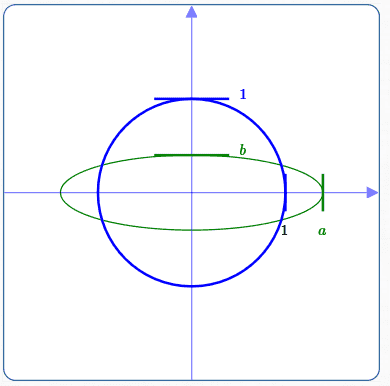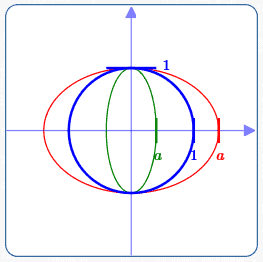﻿ Getting an Ellipse by Stretching/Shrinking a Circle

# Getting an Ellipse by Stretching/Shrinking a Circle

• PRACTICE (online exercises and printable worksheets)

This lesson is optional in the Precalculus course.

This lesson assumes knowledge of equations of ellipses in standard form: foci on the $\,x$-axis.

You may find yourself thinking that an ellipse looks like a ‘deformed circle’:
you know, just grab the ‘ends’ and stretch or compress the circle.
And—you'd be correct, as this lesson shows!

By using earlier work on horizontal and vertical stretching/shrinking of graphs,
we get the equation $\displaystyle\,\frac{x^2}{a^2} + \frac{y^2}{b^2} = 1\,$ easily, as follows:
• Start with the circle of radius $\,1\,$, centered at the origin, which has equation $\,x^2 + y^2 = 1\,$.
• Replace every $\,x\,$ in the circle equation by $\displaystyle\,\frac xa\,$:
$$\begin{gather} \left(\frac xa\right)^2 + y^2 = 1\cr \frac{x^2}{a^2} + y^2 = 1 \end{gather}$$ This moves a point $\,(x,y)\,$ on the circle to a new point $\,(ax,y)\,$ on an ellipse.
For example, the point $\,(1,0)\,$ on the circle moves to $\,(a,0)\,$ on the ellipse.
If $\,a > 1\,$, it is a horizontal stretch.
If $\,0 < a < 1\,$, it is a horizontal shrink.
• Similarly, replace every $\,y\,$ with $\displaystyle\,\frac yb\,$ in the equation $\,x^2 + y^2 = 1\,$, giving the new equation $\displaystyle\,x^2 + \frac{y^2}{b^2} = 1\,$.
This moves a point $\,(x,y)\,$ on the circle to a new point $\,(x,by)\,$ on an ellipse.
For example, the point $\,(0,1)\,$ on the circle moves to $\,(0,b)\,$ on the ellipse.
If $\,b > 1\,$, it is a vertical stretch.
If $\,0 < b < 1\,$, it is a vertical shrink.
• By replacing both $\,x\,$ by $\displaystyle\,\frac xa\,$ and $\,y\,$ by $\displaystyle\,\frac yb\,$, we get the equation $\,\displaystyle \frac{x^2}{a^2} + \frac{y^2}{b^2} = 1\,$.circle of radius $\,1\,$, in blue: $x^2 + y^2 = 1$ ellipse, in green: $$\color{green}{\frac{x^2}{a^2} + \frac{y^2}{b^2} = 1}$$ $\,a > 1\,$, horizontal stretch $\,0 < b < 1\,$, vertical shrinkcircle of radius $\,1\,$, in blue:
$x^2 + y^2 = 1$

horizontal stretch, in red:
$\displaystyle\frac{x^2}{a^2} + y^2 = 1\,$, for $\,a > 1$

horizontal shrink, in green:
$\displaystyle\frac{x^2}{a^2} + y^2 = 1\,$, for $\,0 < a < 1$circle of radius $\,1\,$, in blue:
$x^2 + y^2 = 1$

vertical stretch, in red:
$\displaystyle x^2 + \frac{y^2}{b^2} = 1\,$, for $\,b > 1$

vertical shrink, in green:
$\displaystyle x^2 + \frac{y^2}{b^2} = 1\,$, for $\,0 < b < 1$

## Transformations involving $\,y\,$ for Equations that ARE of the Form $\,y = f(x)\,$

People who have thoroughly studied horizontal and vertical stretching/shrinking
may be a bit perplexed by the treatment of the transformations involving $\,y\,$ in this section.
If this is you, then keep reading!

In that earlier section, we were dealing with a very specific type of equation involving $\,x\,$ and $\,y\,$: an equation of the form $\,y = f(x)\,$.
Such an equation has $\,y\,$ all by itself on one side; the other side involves only $\,x\,$.
For equations of the form $\,y = f(x)\,$, it was easier to treat transformations involving $\,x\,$ and $\,y\,$ differently.
We'll quickly review those differences next.

Transformations involving $y$ for equations of the form $\,y = f(x)\,$:

• Transformations involving $\,y\,$ are intuitive—they work the way you think they should!
• The language used is: ‘DO THIS to the previous $y$-value.’
• EXAMPLE:
Start with the graph of $\,y = x^2\,$.
Multiply each $y$-value of points on the graph by $\,3\,$ (a vertical stretch).
What is the equation of the resulting graph?

$\,y = 3x^2\,$
The previous $y$-values were multiplied by $\,3\,$.
The new $y$-value is $\,3\,$ times the old $y$-value.
Transformations involving $x$ for equations of the form $\,y = f(x)\,$:
• Transformations involving $\,x\,$ are not intuitive—they don't work the way you think they should!
• The language used is: ‘REPLACE the previous $x$-values by ...’
• EXAMPLE:
Start with the graph of $\,y = x^2\,$.
Multiply each $x$-value of points on the graph by $\,3\,$ (a horizontal stretch).
What is the equation of the resulting graph?

$\displaystyle\,y = \left(\frac{x}{3}\right)^2\,$
We replaced every $x$ in the original equation by $\displaystyle\,\frac{x}{3}\,$.
To get the $x$-values multiplied by $\,3\,$ in the graph,
we needed to replace every $\,x\,$ by $\displaystyle\,\frac{x}{3}\,$ ($\,x\,$ divided by $\,3\,$) in the equation!

## Transformations involving $\,y\,$ for Equations that ARE NOT of the Form $\,y = f(x)\,$

Sometimes you need to apply graphical transformations to equations that can't be put in the form $\,y = f(x)\,$.
If the graph of an equation doesn't pass a vertical line test, then it can't be put in the form $\,y = f(x)\,$.

The ellipse equation $\displaystyle\,\frac{x^2}{a^2} + \frac{y^2}{b^2} = 1\,$ is such an equation.
Its graph doesn't pass the vertical line test.
Here, it is possible to solve for $\,y\,$, but it introduces an annoying square root and ‘plus or minus’ sign.

To apply graphical transformations to any equation in $\,x\,$ and $\,y\,$,
(no matter how mixed-up the variables might be),
just use the ‘replace every...’ language for both $\,x\,$ and $\,y\,$.
(Then, both cases are equally counter-intuitive!)

For example:
If we want every $y$-value in a graph to be multiplied by $\,3\,$,
then replace every $\,y\,$ in the original equation by $\,y\,$ divided by $\,3\,$.

This more general technique certainly works in the ‘$\,y = f(x)\,$’ case, but isn't needed:

EXAMPLE:
Start with the graph of $\,y = x^2\,$.
Multiply each $y$-value of points on the graph by $\,3\,$ (a vertical stretch).
What is the equation of the resulting graph?

$\displaystyle\,\frac{y}{3} = x^2\,$
Replace every $\,y\,$ by $\,\frac{y}{3}\,$.
Of course, this gives the same result—just multiply both sides by $\,3\,$ to get $\,y = 3x^2\,$!

Master the ideas from this section

When you're done practicing, move on to:
(optional) two useful transforms:
reflection about the line $\,y = x\,$ and
counterclockwise rotation by $\,90^\circ$